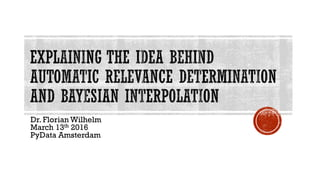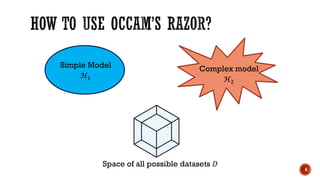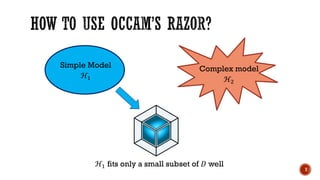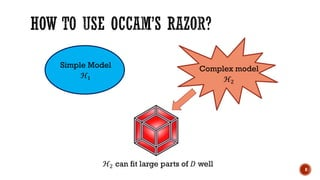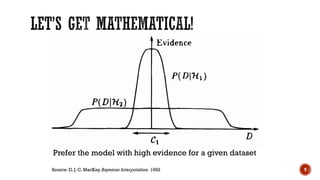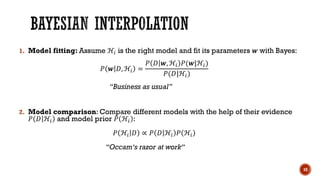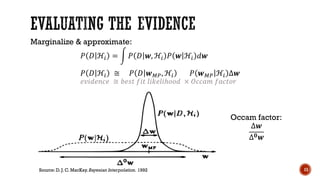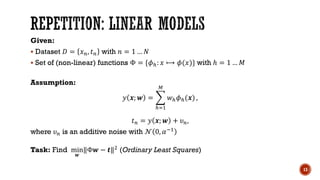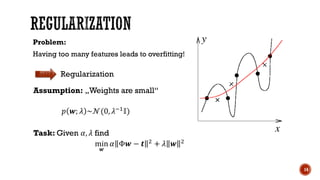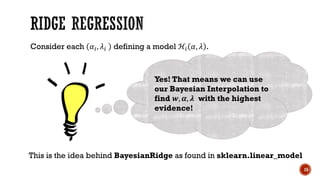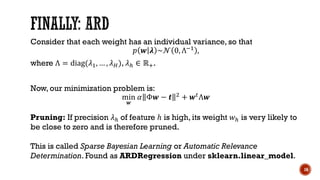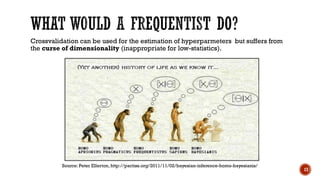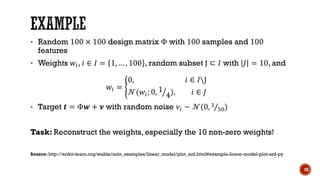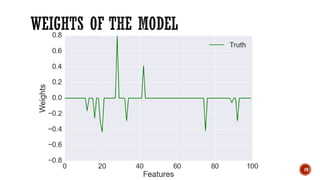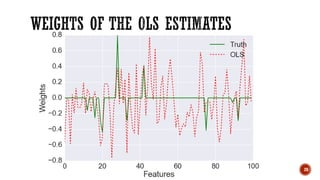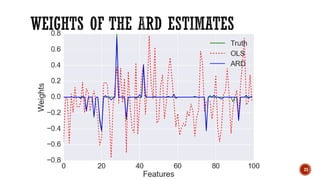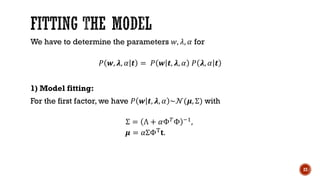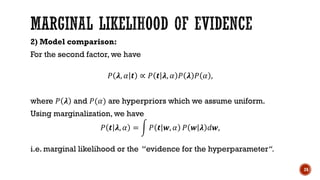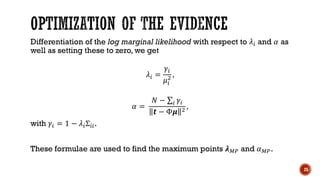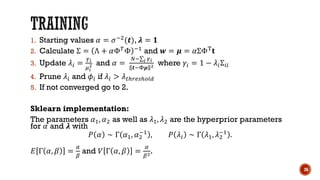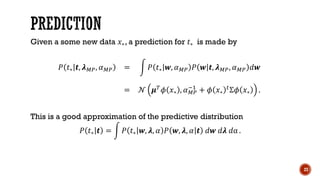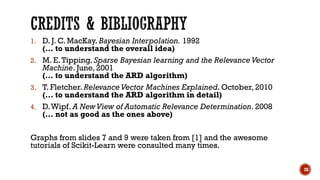1 of 29

## RecommendedAlgorithms DMRokonuzzaman RonyLecture 9 PerceptronMarina SantiniPRML Chapter 1Sunwoo KimPRML 条件付き混合モデル 14.5tmtm otmNeural networks introductionآيةالله عبدالحكيمPRML Chapter 5Sunwoo Kim

### Explaining the idea behind automatic relevance determination and bayesian interpolation

1. Dr. Florian Wilhelm March 13th 2016 PyData Amsterdam P 1
2. 2 What‘s the best model to describe our data? And what does „best“ actually mean?
3. 3
4. 4
5. 5 Simple model „Generality“ Complex model „Best Fit“ Occam‘s Razor: „It is vain to do with more what can be done with fewer“
6. 6 Simple Model ℋ1 Complex model ℋ2 Space of all possible datasets 𝐷
7. 7 Simple Model ℋ1 Complex model ℋ2 ℋ1 fits only a small subset of 𝐷 well
8. 8 Simple Model ℋ1 Complex model ℋ2 ℋ2 can fit large parts of 𝐷 well
9. 9 Prefer the model with high evidence for a given dataset Source: D. J. C. MacKay. Bayesian Interpolation. 1992
10. 1. Model fitting: Assume ℋ𝑖 is the right model and fit its parameters 𝒘 with Bayes: 𝑃 𝒘 𝐷, ℋ𝑖 = 𝑃 𝐷 𝒘, ℋ𝑖 𝑃(𝒘|ℋ𝑖) 𝑃(𝐷|ℋ𝑖) “Business as usual” 2. Model comparison: Compare different models with the help of their evidence 𝑃 𝐷 ℋ𝑖 and model prior 𝑃 ℋ𝑖 : 𝑃 ℋ𝑖 𝐷 ∝ 𝑃 𝐷 ℋ𝑖 𝑃 ℋ𝑖 “Occam‘s razor at work“ 10
11. Marginalize & approximate: 𝑃 𝐷 ℋ𝑖 = 𝑃 𝐷 𝒘, ℋ𝑖 𝑃 𝒘 ℋ𝑖 𝑑𝒘 𝑃 𝐷 ℋ𝑖 ≅ 𝑃 𝐷 𝒘 𝑀𝑃, ℋ𝑖 𝑃 𝒘 𝑀𝑃 ℋ𝑖 ∆𝒘 𝑒𝑣𝑖𝑑𝑒𝑛𝑐𝑒 ≅ 𝑏𝑒𝑠𝑡 𝑓𝑖𝑡 𝑙𝑖𝑘𝑒𝑙𝑖ℎ𝑜𝑜𝑑 × 𝑂𝑐𝑐𝑎𝑚 𝑓𝑎𝑐𝑡𝑜𝑟 11 Occam factor: ∆𝒘 ∆ 𝟎 𝒘 Source: D. J. C. MacKay. Bayesian Interpolation. 1992
12. 12
13. Given:  Dataset 𝐷 = 𝑥 𝑛, 𝑡 𝑛 with 𝑛 = 1 … 𝑁  Set of (non-linear) functions Φ = {𝜙ℎ: 𝑥 ⟼ 𝜙(𝑥)} with ℎ = 1 … 𝑀 Assumption: 𝑦 𝒙; 𝒘 = ℎ=1 𝑀 𝑤ℎ 𝜙ℎ(𝒙) , 𝑡 𝑛 = 𝑦 𝒙; 𝒘 + 𝜐 𝑛, where 𝜐 𝑛 is an additive noise with 𝒩 0, 𝛼−1 Task: Find min 𝒘 ‖Φ𝒘 − 𝒕‖2 (Ordinary Least Squares) 13
14. 14 Problem: Having too many features leads to overfitting! Regularization Assumption: „Weights are small“ 𝑝 𝒘; 𝜆 ~𝒩(0, 𝜆−1 𝕀) Task: Given 𝛼, 𝜆 find min 𝒘 𝛼 Φ𝒘 − 𝒕 2 + 𝜆 𝒘 2
15. 15 Consider each 𝛼𝑖, 𝜆𝑖 defining a model ℋ𝑖 𝛼, 𝜆 . Yes! That means we can use our Bayesian Interpolation to find 𝒘, 𝜶, 𝝀 with the highest evidence! This is the idea behind BayesianRidge as found in sklearn.linear_model
16. Consider that each weight has an individual variance, so that 𝑝 𝒘 𝝀 ~𝒩 0, Λ−1 , where Λ = diag(𝜆1, … , 𝜆 𝐻), 𝜆ℎ ∈ ℝ+. Now, our minimization problem is: min 𝒘 𝛼 Φ𝒘 − 𝒕 2 + 𝒘 𝑡Λ𝒘 16 Pruning: If precision 𝜆ℎ of feature ℎ is high, its weight 𝑤ℎ is very likely to be close to zero and is therefore pruned. This is called Sparse Bayesian Learning or Automatic Relevance Determination. Found as ARDRegression under sklearn.linear_model.
17. Crossvalidation can be used for the estimation of hyperparmeters but suffers from the curse of dimensionality (inappropriate for low-statistics). 17 Source: Peter Ellerton, http://pactiss.org/2011/11/02/bayesian-inference-homo-bayesianis/
18. • Random 100 × 100 design matrix Φ with 100 samples and 100 features • Weights 𝑤𝑖, 𝑖 ∈ 𝐼 = 1, … , 100 , random subset J ⊂ 𝐼 with 𝐽 = 10, and 𝑤𝑖 = 0, 𝑖 ∈ 𝐼J 𝒩(𝑤𝑖; 0, 1 4), 𝑖 ∈ 𝐽 • Target 𝒕 = Φ𝒘 + 𝝂 with random noise 𝜈𝑖 ∼ 𝒩(0, 1 50) Task: Reconstruct the weights, especially the 10 non-zero weights! Source: http://scikit-learn.org/stable/auto_examples/linear_model/plot_ard.html#example-linear-model-plot-ard-py 18
19. 19
20. 20
21. 21
22. 22
23. We have to determine the parameters 𝑤, 𝜆, 𝛼 for 𝑃 𝒘, 𝝀, 𝛼 𝒕 = 𝑃 𝒘 𝒕, 𝝀, 𝛼 𝑃 𝝀, 𝛼 𝒕 1) Model fitting: For the first factor, we have 𝑃 𝒘 𝒕, 𝝀, 𝛼 ~𝒩(𝝁, Σ) with Σ = Λ + 𝛼Φ 𝑇 Φ −1 , 𝝁 = 𝛼ΣΦT 𝐭. 23
24. 2) Model comparison: For the second factor, we have 𝑃 𝝀, 𝛼 𝒕 ∝ 𝑃 𝒕 𝝀, 𝛼 𝑃 𝝀 𝑃 𝛼 , where 𝑃 𝝀 and 𝑃(𝛼) are hyperpriors which we assume uniform. Using marginalization, we have 𝑃 𝒕 𝝀, 𝛼 = 𝑃 𝒕 𝒘, 𝛼 𝑃 𝒘 𝝀 𝑑𝒘, i.e. marginal likelihood or the “evidence for the hyperparameter“. 24
25. Differentiation of the log marginal likelihood with respect to 𝜆𝑖 and 𝛼 as well as setting these to zero, we get 𝜆𝑖 = 𝛾𝑖 𝜇𝑖 2 , 𝛼 = 𝑁 − 𝑖 𝛾𝑖 𝒕 − Φ𝝁 2 , with 𝛾𝑖 = 1 − 𝜆𝑖Σ𝑖𝑖. These formulae are used to find the maximum points 𝝀 𝑀𝑃 and 𝛼 𝑀𝑃. 25
26. 1. Starting values 𝛼 = 𝜎−2(𝒕), 𝝀 = 𝟏 2. Calculate Σ = Λ + 𝛼Φ 𝑇Φ −1 and 𝒘 = 𝝁 = 𝛼ΣΦT 𝐭 3. Update 𝜆𝑖 = 𝛾 𝑖 𝜇 𝑖 2 and 𝛼 = 𝑁− 𝑖 𝛾 𝑖 𝒕−Φ𝝁 2 where 𝛾𝑖 = 1 − 𝜆𝑖Σ𝑖𝑖 4. Prune 𝜆𝑖 and 𝜙𝑖 if 𝜆𝑖 > 𝜆 𝑡ℎ𝑟𝑒𝑠ℎ𝑜𝑙𝑑 5. If not converged go to 2. Sklearn implementation: The parameters 𝛼1, 𝛼2 as well as 𝜆1, 𝜆2 are the hyperprior parameters for 𝛼 and 𝝀 with 𝑃 𝛼 ∼ Γ 𝛼1, 𝛼2 −1 , 𝑃 𝜆𝑖 ∼ Γ 𝜆1, 𝜆2 −1 . 𝐸 Γ 𝛼, 𝛽 = 𝛼 𝛽 and 𝑉 Γ 𝛼, 𝛽 = 𝛼 𝛽2. 26
27. Given a some new data 𝑥∗, a prediction for 𝑡∗ is made by 𝑃 𝑡∗ 𝒕, 𝝀 𝑀𝑃, 𝛼 𝑀𝑃 = 𝑃 𝑡∗ 𝒘, 𝛼 𝑀𝑃 𝑃 𝒘 𝒕, 𝝀 𝑀𝑃, 𝛼 𝑀𝑃 𝑑𝒘 = 𝒩 𝝁 𝑇 𝜙 𝑥∗ , 𝛼 𝑀𝑃 −1 + 𝜙 𝑥∗ 𝑡Σ𝜙 𝑥∗ . This is a good approximation of the predictive distribution 𝑃 𝑡∗ 𝒕 = 𝑃 𝑡∗ 𝒘, 𝝀, 𝛼 𝑃 𝒘, 𝝀, 𝛼 𝒕 𝑑𝒘 𝑑𝝀 𝑑α . 27
28. 1. D. J. C. MacKay. Bayesian Interpolation. 1992 (… to understand the overall idea) 2. M. E.Tipping. Sparse Bayesian learning and the RelevanceVector Machine. June, 2001 (… to understand the ARD algorithm) 3. T. Fletcher. RelevanceVector Machines Explained. October, 2010 (… to understand the ARD algorithm in detail) 4. D.Wipf. A NewView of Automatic Relevance Determination. 2008 (… not as good as the ones above) Graphs from slides 7 and 9 were taken from  and the awesome tutorials of Scikit-Learn were consulted many times. 28
29. 29